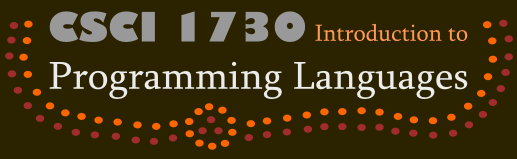### Problem 1

Consider a datatype definition that introduces numeric lists as the sum of two variants: `nempty` and `ncons`. This defines a new type (`nlist`), and each constructor (such as `ncons`) creates a value of that type. As a result, however, selectors (such as `nfirst`) cannot statically determine whether or not they have been given the correct variant of the datatype, and must rely on a check from the run-time system.

On the Web, you read a proposal for a better design: Suppose, instead, each variant creates a new type. Then you can give a precise type for the selector (e.g., above, `nfirst` would take only `ncons` values, not `nempty` or `nlist` ones), thus turning the dynamic check into a static one, thereby increasing the effectiveness of the type system. Because this was proposed in Twitter, it's frustratingly short on details.

Write at most a page (of reasonably-sized text) exploring this design. Can we build an effective type system out of this idea? If so, how, and if not, why not? If we can't as stated, can we turn this into something that actually works? Have you seen any languages that do something like this? Solutions to this problem which are much shorter than one page are certainly acceptable so long as they get the point across.

### Problem 2

Consider the program:
`(+ 1 (first (cons true empty)))`
This program has a type error.

Generate constraints for this program. Isolate the smallest set of these constraints that, solved together, identify the type error.

Feel free to label the sub-expressions above with superscripts for use when writing and solving constraints.

### Problem 3

Consider the following typed expression:

```{fun {f : B1 } : B2
{fun {x : B3 } : B4
{fun {y : B5 } : B6
{cons x {f {f y}}}}}}```

We have left the types unspecified (`Bn`) to be filled in by the type inference process. Derive type constraints from the above program. Then solve these constraints. From these solutions, fill in the values of the boxes. Be sure to show all the steps specified by the algorithms (i.e., writing the answer based on intuition or knowledge is insufficient). You should use type variables where necessary. To save writing, you can annotate each expression with an appropriate type variable, and present the rest of the algorithm in terms of these type variables alone (to avoid having to copy the corresponding expressions). If you do this, be sure to annotate every sub-expression with a type variable. Be sure the annotations are clearly readable!

### Problem 4

The textbook notes that recursive types enable us to type ω (and, by extension, Ω). Provide a type for the function ω, and discuss informally how type-checking would work with the type you describe to bless an application of ω to itself.

Reminder: we defined ω as `(lambda (x) (x x))`, and Ω as the application of ω to itself: `((lambda (x) (x x)) (lambda (x) (x x))).`

### Problem 5

Because we introduced this problem after the original problem set went out, you are not required to do it. You may do it for bonus points, or you may do it in place of one of the other three problems.

In Java, there is no single type for numbers, and `float`, `int`, etc., are all distinct types and very few of these can be directly substituted for others. At the other extreme, in Typed Racket, both `Integer <: Number` and `Float <: Number`, and indeed there is a large and complex subtype hierarchy of numbers (including types such as `Nonpositive-Float` and even `Positive-Byte`) that you can look up in its documentation.

What are the tradeoffs between these two designs?

### Handin

Put your solution to each problem in a separate text file labeled {1, 2, 3, 4}.txt. If you do the fifth problem, you may turn in 5.txt and/or leave out one of the first four files.

From the directory containing the files for this assignment, execute

`/course/cs173/bin/cs173handin types-writ`